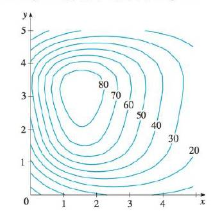Chapter 14, Problem 8RE

Chapter
Section
Textbook Problem

The contour map of a function f is shown, (a) Estimate the value of f(3, 2).(b) Is fx(3, 2) positive or negative? Explain.(c) Which is greater, fy(2, 1) or fy(2, 2)? Explain.(a)

To determine

To estimate: The value of f(3,2) from the given contour map of the functionf.

Explanation

It is observed from the given contour map that the point (3,2) lies between the level curves z of values 50 and 60

(b)

To determine

To explain: The sign of fx(3,2) from the given contour map of the function f.

(c)

To determine

To explain: Which function value is higher fy(2,1)orfy(2,2) .

Still sussing out bartleby?

Check out a sample textbook solution.

See a sample solution

The Solution to Your Study Problems

Bartleby provides explanations to thousands of textbook problems written by our experts, many with advanced degrees!

Get Started

Calculate y'. 18. y = cot(csc x)

Single Variable Calculus: Early Transcendentals, Volume I

Find the median for the following set of scores: 1, 9, 3, 6, 4, 3, 11, 10

Essentials of Statistics for The Behavioral Sciences (MindTap Course List)

Use the simplex method in Problems 11-14. 13. Minimize subject to

Mathematical Applications for the Management, Life, and Social Sciences

Evaluate the integral, if it exists. 12(8x3+3x2)dx

Single Variable Calculus: Early Transcendentals

Using for |x| < 1 and differentiation, find a power series for .

Study Guide for Stewart's Multivariable Calculus, 8th